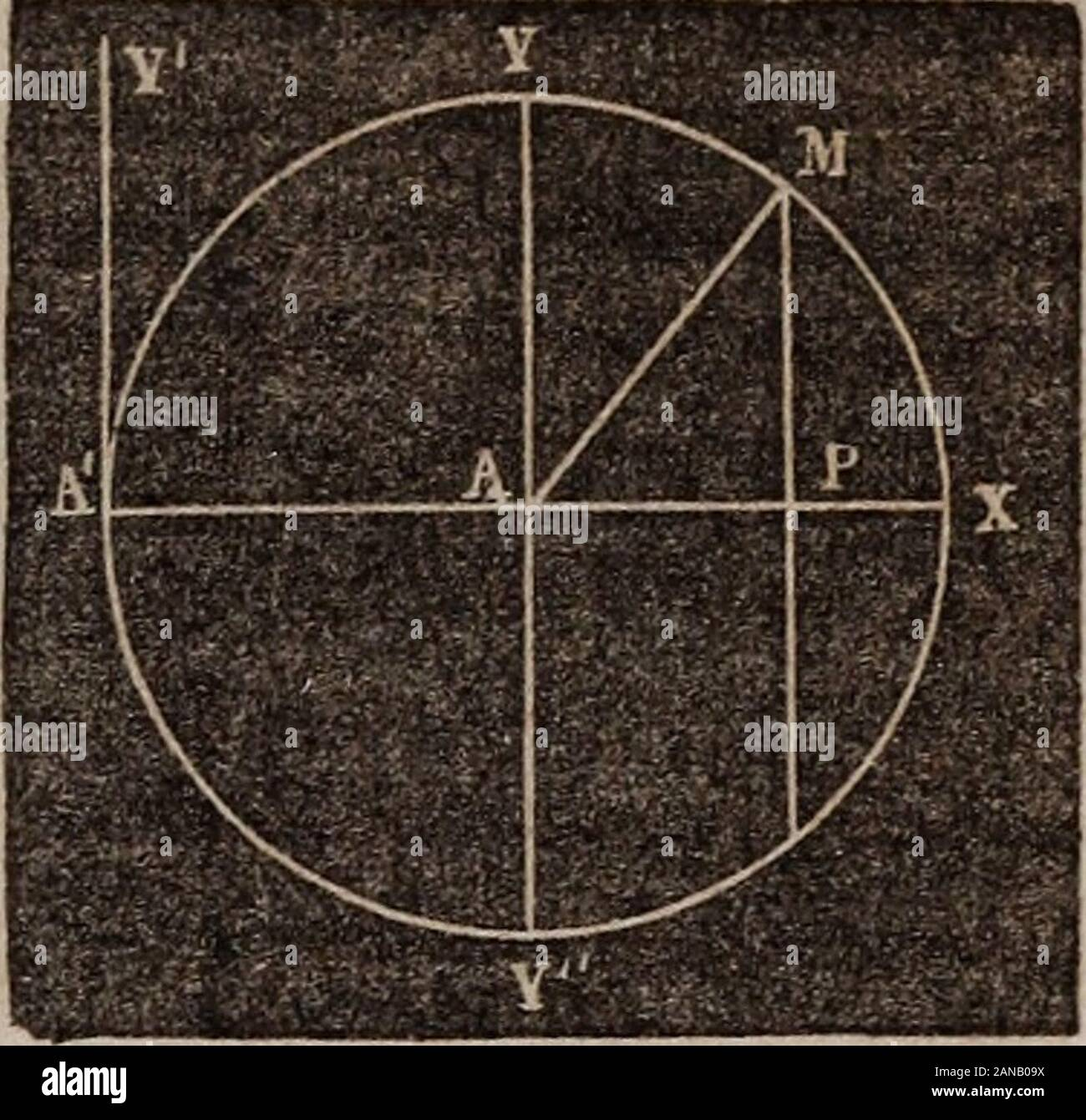# Elements of analytical geometry and the differential and integral calculus . erred toco-ordinates requires equations of the second degree. PROPOSITION I.7h find the equation of the circle. Let the origin be the center ofthe circle. Draw AM.o any pointin the circumference, and let fallMP perpendicular to the axis of X.Put AP=x, PM=:y and AM=P. Then the right angled triangle^PJf gives x^+y=P^ (1) and this is the equation of the circlewhen the zero point is the center. When y=:0, x^ = R^, or ±:x=P, that is, Pis at X or AWhenar=0, y=R^, or ±y=R, showing that if on the cir-cumference is then at Y## Image details

Contributor:

The Reading Room / Alamy Stock Photo

Image ID:

2ANB09X

File size:

Releases:

Model - no | Property - noDo I need a release?

Dimensions:

1611 x 1551 px | 27.3 x 26.3 cm | 10.7 x 10.3 inches | 150dpi